Courses

# Inverse of a Matrix - Matrices and Determinants, Business Mathematics & Statistics B Com Notes | EduRev

## B Com : Inverse of a Matrix - Matrices and Determinants, Business Mathematics & Statistics B Com Notes | EduRev

The document Inverse of a Matrix - Matrices and Determinants, Business Mathematics & Statistics B Com Notes | EduRev is a part of the B Com Course Business Mathematics and Statistics.
All you need of B Com at this link: B Com

INVERSE OF A MATRIX
Minors and Cofactors of the elements of a determinant.
The minor of an element aij of a determinant A is denoted by Mij and is the determinant obtained from A by deleting the row and the column where aij occurs.
The cofactor of an element aij with minor Mij is denoted by Cij and is defined as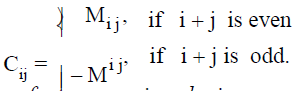Thus, cofactors are signed minors.

In the case of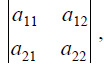, we have

M11 = a 22 , M12 = a 21, M21 = a , , M22 = a11
Also C11 = a22 , C12 = -a21, C21 = -a12 , C22 = a11

In the case of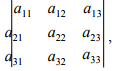we have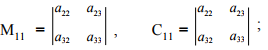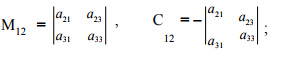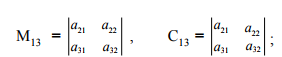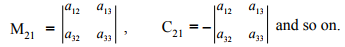The transpose of the matrix got by replacing all the elements of a square matrix A by their corresponding cofactors in | A | is called the Adjoint of A or Adjugate of A and is denoted by Adj A.

Thus, AdjA =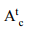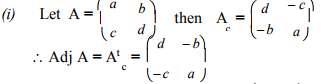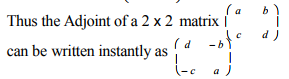(ii) Adj I = I, where I is the unit matrix.
(v) If A is a square matrix of order 2, then |AdjA| = |A|
If A is a square matrix of order 3, then |Adj A| = |A|2

Example 1 Write the Adjoint of the matrix A =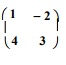Solution :
Adj A =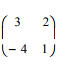Example 2
Find the Adjoint of the matrix A =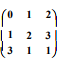Solution :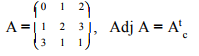Now,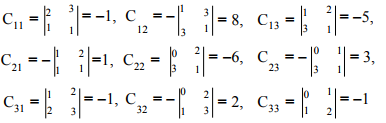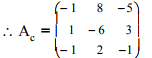Hence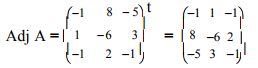Inverse of a non singular matrix.
The inverse of a non singular matrix A is the matrix B such that AB = BA = I. B is then called the inverse of A and denoted by A-1 .
Note
(i) A non square matrix has no inverse.
(ii) The inverse of a square matrix A exists only when |A| ≠ 0 that is, if A is a singular matrix then A-1 does not exist.
(iii) If B is the inverse of A then A is the inverse of B. That is B = A-1 ⇒ A = B-1.
(iv) A A-1 = I = A-1 A
(v) The inverse of a matrix, if it exists, is unique. That is, no matrix can have more than one inverse.
(vi) The order of the matrix A-1 will be the same as that of A.
(vii) I-1 = I
(viii) (AB)-1 = B-1 A-1 , provided the inverses exist.
(ix) A2 = I implies A-1 = A
(x) If AB = C then (a) A = CB-1 (b) B = A-1C, provided the inverses exist.
(xi) We have seen that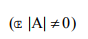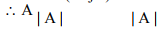This suggests that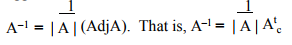(xii) (A-1 ) -1 = A, provided the inverse exists.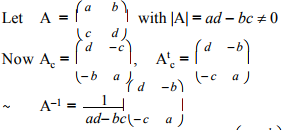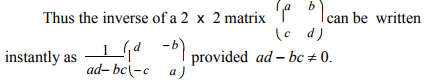Example 3​
Find the inverse of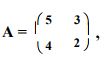if it exists

Solution :​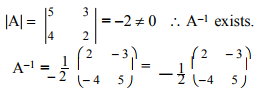Example 4
Show that the inverses of the following do not exist :​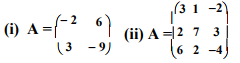Solution :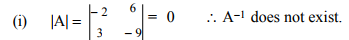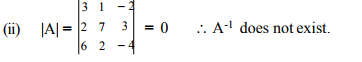Example 5
Find the inverse of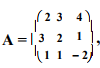if it exists.

Solution :​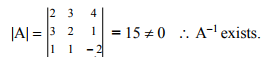We have,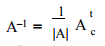Now, the cofactors are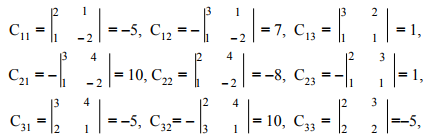Hence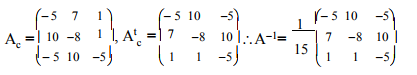Example 6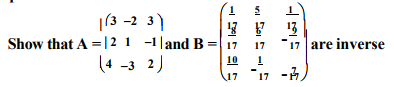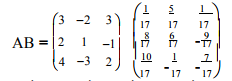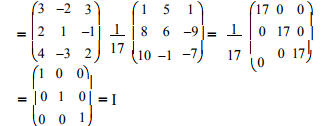Since A and B are square matrices and AB = I, A and B are inverse of each other

Offer running on EduRev: Apply code STAYHOME200 to get INR 200 off on our premium plan EduRev Infinity!

122 videos|142 docs

,

,

,

,

,

,

,

,

,

,

,

,

,

,

,

,

,

,

,

,

,

,

,

,

;